# Class 11 Maths NCERT Solutions for Chapter 15 Statistics Exercise 15.1### Statistics Exercise 15.1 Solutions

1. Find the mean deviation about the mean for the data
4, 7, 8, 9, 10, 12, 13, 17

Solution

The given data is
4, 7, 8, 9, 10, 12, 13, 17
Mean of the data,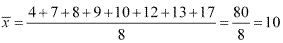The deviations of the respective observations from the mean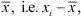are –6, –3, –2, –1, 0, 2, 3, 7
The absolute values of the deviations, i.e.,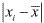are 6, 3, 2, 1, 0, 2, 3, 7
The required mean deviation about the mean is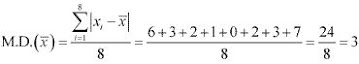2. Find the mean deviation about the mean for the data
38, 70, 48, 40, 42, 55, 63, 46, 54, 44

Solution

The given data is
38, 70, 48, 40, 42, 55, 63, 46, 54, 44
Mean of the given data,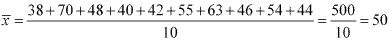The deviations of the respective observations from the meanare -12, 20, -2, -10, -8, 5, 13, -4, 4, -6
The absolute values of the deviations, i.e.,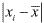, are 12, 20, 2, 10, 8, 5, 13, 4, 4, 6.
The required mean deviation about the mean is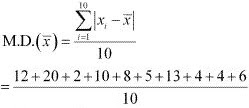= 84/10
= 8.4

3. Find the mean deviation about the median for the data.
13, 17, 16, 14, 11, 13, 10, 16, 11, 18, 12, 17

Solution

The given data is
13, 17, 16, 14, 11, 13, 10, 16, 11, 18, 12, 17
Here, the numbers of observations are 12, which is even.
Arranging the data in ascending order, we obtain
10, 11, 11, 12, 13, 13, 14, 16, 16, 17, 17, 18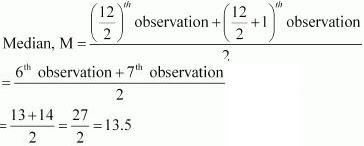The deviations of the respective observations from the median, i.e. xi – M ,are
–3.5, –2.5, –2.5, –1.5, –0.5, –0.5, 0.5, 2.5, 2.5, 3.5, 3.5, 4.5
The absolute values of the deviations, |xi – M |, are
3.5, 2.5, 2.5, 1.5, 0.5, 0.5, 0.5, 2.5, 2.5, 3.5, 3.5, 4.5
The required mean deviation about the median is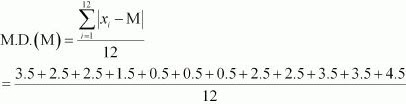= 28/12 = 2.33

4. Find the mean deviation about the median for the data
36, 72, 46, 42, 60, 45, 53, 46, 51, 49

Solution

The given data is
36, 72, 46, 42, 60, 45, 53, 46, 51, 49
Here, the number of observations is 10, which is even.
Arranging the data in ascending order, we obtain
36, 42, 45, 46, 46, 49, 51, 53, 60, 72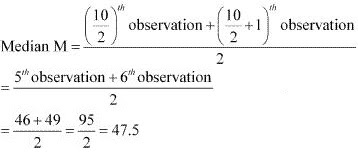The deviations of the respective observations from the median, i.e. xi – M are
–11.5, –5.5, –2.5, –1.5, –1.5, 1.5, 3.5, 5.5, 12.5, 24.5
The absolute values of the deviations,  |xi – M |, are
11.5, 5.5, 2.5, 1.5, 1.5, 1.5, 3.5, 5.5, 12.5, 24.5
Thus, the required mean deviation about the median is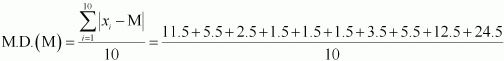= 70/10 = 7

5. Find the mean deviation about the mean for the data.

 xi 5 10 15 20 25 fi 7 4 6 3 5
Solution
 xi fi fixi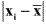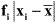5 7 35 9 63 10 4 40 4 16 15 6 90 1 6 20 3 60 6 18 25 5 125 11 55 25 350 158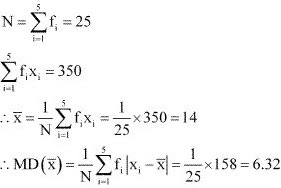6. Find the mean deviation about the mean for the data

 xi 10 30 50 70 90 fi 4 24 28 16 8

Solution

 xi fi fixi10 4 40 40 160 30 24 720 20 480 50 28 1400 0 0 70 16 1120 20 320 90 8 720 40 320 80 4000 1280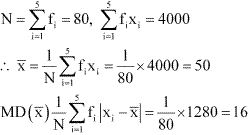7. Find the mean deviation about the median for the data.
 xi 5 7 9 10 12 15 fi 8 6 2 2 2 6

Solution

The given observations are already in ascending order.
Adding a column corresponding to cumulative frequencies of the given data, we obtain the following table.

 xi fi­ c.f. 5 8 8 7 6 14 9 2 16 10 2 18 12 2 20 15 6 26

Here, N = 26, which is even.
Median is the mean of 13th and 14th observations. Both of these observations lie in the cumulative frequency 14, for which the corresponding observation is 7.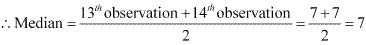The absolute values of the deviations from median, i.e, |xi – M|, are

 |xi – M| 2 0 2 3 5 8 fi 8 6 2 2 2 6 fi |xi – M| 16 0 4 6 10 48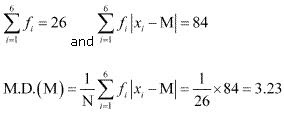8. Find the mean deviation about the median for the data
 xi 15 21 27 30 35 fi 3 5 6 7 8
Solution
The given observations are already in ascending order.
Adding a column corresponding to cumulative frequencies of the given data, we obtain the following table.
 xi fi c.f. 15 3 3 21 5 8 27 6 14 30 7 21 35 8 29
Here, N = 29, which is odd.
∴ Median = [(29 + 1)/2 ]th observation = 15th observation
This observation lies in the cumulative frequency 21, for which the corresponding observation is  30.
Median = 30
The absolute values of the deviations from median, i.e. |xi – M|, are
 |xi – M| 15 9 3 0 5 fi 3 5 6 7 8 fi |xi – M| 45 45 18 0 40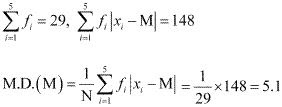9. Find the mean deviation about the mean for the data.
 Income per day Number of persons 0-100 4 100-200 8 200-300 9 300-400 10 400-500 7 500-600 5 600-700 4 700-800 3
Solution
The following table is formed.
 Income per day Number of person fi Mid – point xi fi xi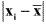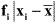0 – 100 4 50 200 308 1232 100 – 200 8 150 1200 208 1664 200 – 300 9 250 2250 108 972 300 – 400 10 350 3500 8 80 400 – 500 7 450 3150 92 644 500 – 600 5 550 2750 192 960 600 – 700 4 650 2600 292 1168 700 – 800 3 750 2250 392 1176 50 17900 7896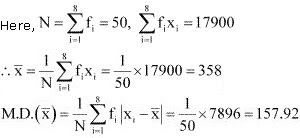10. Find the mean deviation about the mean for the data
 Height in cms Number of boys 95-105 9 105-115 13 115-125 26 125-135 30 135-145 12 145-155 10
Solution
The following table is formed.
 Height in cms Number of boys fi Mid – point xi fi xi 95 – 105 9 100 900 25.3 227.7 105 – 115 13 110 1430 15.3 198.9 115 – 125 26 120 3120 5.3 137.8 125 – 135 30 130 3900 4.7 141 135 – 145 12 140 1680 14.7 176.4 145 – 155 10 150 1500 24.7 247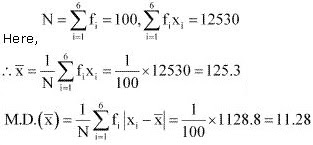11. Find the mean deviation about median for the following data:
 Marks Number of girls 0-10 6 10-20 8 20-30 14 30-40 16 40-50 4 50-60 2
Solution
The following table is formed.
 Marks Number of boys  fi Cumulative frequency (c. f.) Mid – point xi |xi – Med.| fi |xi – Med.| 0 – 10 6 6 5 22.85 137.1 10 – 20 8 14 15 12.85 102.8 20 – 30 14 28 25 2.85 39.9 30 – 40 16 44 35 7.15 114.4 40 – 50 4 48 45 17.15 68.6 50 – 60 2 50 55 27.15 54.3 50 517.1

The class interval containing the (N/2)th or 25th item is  20 - 30 .
Therefore, 20 - 30  is the median class.
It is known that,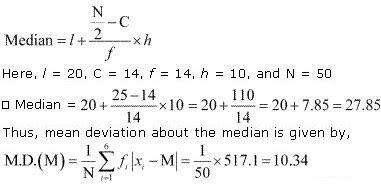12. Calculate the mean deviation about median age for the age distribution of 100 persons given below:
 Age Number 16-20 5 21-25 6 26-30 12 31-35 14 36-40 26 41-45 12 46-50 16 51-55 9
Solution
The given data is not continuous. Therefore, it has to be converted into continuous frequency distribution by subtracting 0.5 from the lower limit and adding 0.5 to the upper limit of each class interval.
The table is formed as follows.
 Age Number fi Cumulative frequency (c.f.) Mid – point xi |xi – Med.| fi |xi – Med.| 15.5 – 20.5 5 5 18 20 100 20.5 – 25.5 6 11 23 15 90 25.5 – 30.5 12 23 28 10 120 30.5 – 35.5 14 37 33 5 70 35.5 – 40.5 26 63 37 0 0 40.5 – 45.5 12 75 43 5 60 45.5 – 50.5 16 91 48 10 160 50.5 – 55.5 9 100 53 15 135 100 735
The class interval containing the (N/2)th or  50th item is  35.5 - 40.5 .
Therefore, 35.5 - 40.5 is the median class.
It is known that,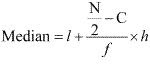Here, l = 35.5 , C = 37 , F = 26, h = 5, and N = 100